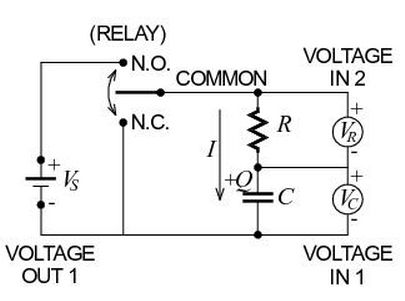# Problem: True or FalseThe transient currents that flow in this experiment decay exponentially to zero.

###### FREE Expert Solution

The current while the capacitor charges is given by

$\overline{){\mathbit{I}}{\mathbf{=}}\frac{{\mathbf{V}}_{\mathbf{b}}}{\mathbf{R}}{{\mathbf{e}}}^{\mathbf{-}\mathbf{\left(}\mathbf{t}\mathbf{/}\mathbf{RC}\mathbf{\right)}}}$

Vb is the battery voltage.

The current while the capacitor discharges is given by

$\overline{){\mathbf{I}}{\mathbf{=}}\frac{{\mathbf{V}}_{\mathbf{0}}}{\mathbf{R}}{{\mathbf{e}}}^{\mathbf{-}\mathbf{\left(}\mathbf{t}\mathbf{/}\mathbf{RC}\mathbf{\right)}}}$

86% (430 ratings)###### Problem DetailsTrue or False

The transient currents that flow in this experiment decay exponentially to zero.

Frequently Asked Questions

What scientific concept do you need to know in order to solve this problem?

Our tutors have indicated that to solve this problem you will need to apply the !! Resistor-Capacitor Circuits concept. If you need more !! Resistor-Capacitor Circuits practice, you can also practice !! Resistor-Capacitor Circuits practice problems.